Courses

# Travelling Salesman Problem (TSP) Computer Science Engineering (CSE) Notes | EduRev

## Computer Science Engineering (CSE) : Travelling Salesman Problem (TSP) Computer Science Engineering (CSE) Notes | EduRev

The document Travelling Salesman Problem (TSP) Computer Science Engineering (CSE) Notes | EduRev is a part of the Computer Science Engineering (CSE) Course Theory of Computation.
All you need of Computer Science Engineering (CSE) at this link: Computer Science Engineering (CSE)

Part VIII. Travelling Salesman Problem (TSP)

12 Travelling Salesman Problem (TSP)

Travelling Salesman
A salesman begins his tour from a city. He visits a set of cities and he finishes at the city he starts from. Each city must be visited exactly once.

Travelling Salesman Problem (TSP)
A graph of a set of cities is given. A salesman starts from a city. He must visit each city exactly once and should finish at the city he starts from. The path he follows should be the shortest one or should have a cost value less than k.Thsu TSP is a special case of Hamiltonian cycle problem where the path cost should be minimum.
Consider the following graph containing cities u, v, w, x.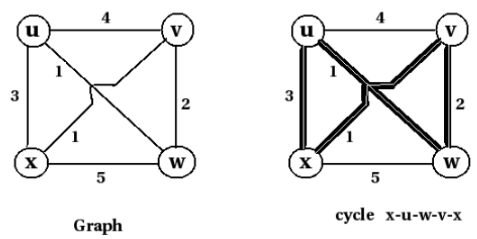Here the traveling salesman can follow the path x-u-w-v-x which forms the shortest path with cost 7km.

TSP is NP-Complete
We know that a problem is in class NP-Complete if: it is in NP and it is NP Hard.

Theorem:
TSP is NP-complete.

Proof:

Step 1: Prove that TSP is in class NP.
A set with n elements has 2n possible subsets. Then a graph with n vertices has 2n possible permutations of vertices.So an algorithm needs to check whether any one of these permutation form a hamiltonian path with cost value less than k. If the graph is represented as an adjacency matrix, it will take n! comparisons. It has worst case time complexity
Θ(2√n). (not polynomial time, but exponential time). Let we are given a graph and a ’certificate’ telling that a sequence of vertices form a hamiltonian cycle with cost value
less than k. An algorithm can verify whether this cycle has cost less than k in polynomial time. So TSP is in class NP.

Step 2: Prove that hamiltonian cycle problem is NP-hard.
We know that a problem is NP-Hard, if every problem in NP can be reduced to this problem in polynomial time.The approach we used is as follows:
In the previous section, we already proved that all NP problems can be reduced to SAT. Then, again we found that SAT problem can be reduced to 3-CNF-SAT problem. Again, we reduced 3-CNF-SAT problem to clique problem. Again, we reduced clique problem to vertex cover problem. Again we reduced vertex cover problem to hamiltonian cycle problem.
Then, if we can reduce hamiltonian cycle problem to TSP in polynomial time, we can say that TSP is NP-hard.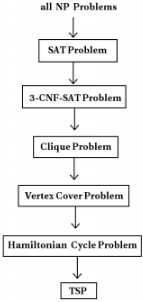We need to reduce hamiltonian cycle problem to TSP.
Consider a graph,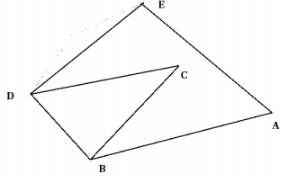Following is an instance of hamiltonian cycle.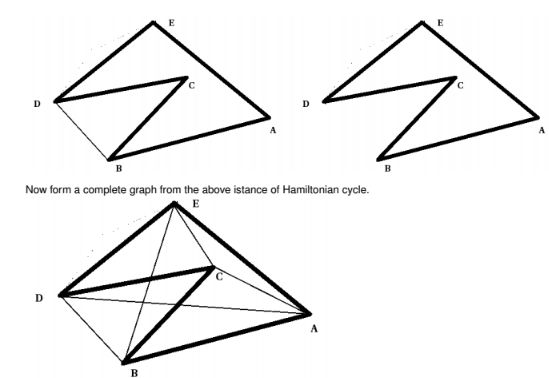Next we assign cost value to every edge of this graph. If the edge is present in hamiltonian cycle, assign cost value 0 to it. If it is not, assign cost value 1 to it.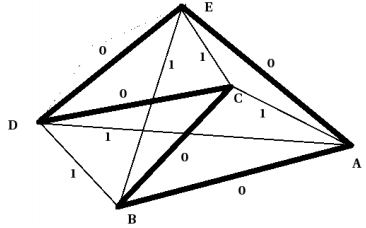From this, an instance of TSP can be produced from the above graph by traversing through the edges which have cost value 0.
Thus hamiltonian cycle problem is reduced to TSP. So TSP is NP-hard.

In step 1, we proved that TSP is in NP.
In step 2, we proved that TSP is NP-hard.
So, TSP is an NP-complete problem.

Offer running on EduRev: Apply code STAYHOME200 to get INR 200 off on our premium plan EduRev Infinity!

## Theory of Computation

18 videos|43 docs|39 tests

,

,

,

,

,

,

,

,

,

,

,

,

,

,

,

,

,

,

,

,

,

;